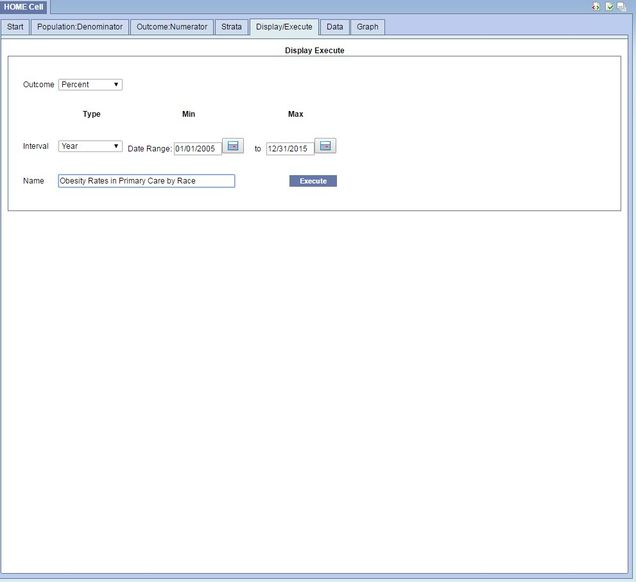# Display/Execute

The Display/Execute tab allows the user to: 1)  select the format (count, proportion, average) for the outcome of interest; 2) configure the reference interval type (month, year, full time period); 3) set a start and end date for the period; and 4) for count and average measures to specify the concept to be counted or averaged.

• Percent calculates the proportion of patients with the outcome by dividing the numerator by the denominator for each strata.
• Count calculates the number of times the concept is observed for persons in the numerator and denominator populations for each strata.
• Average calculates the average value of the concept for persons in the numerator and denominator populations for each strata.

After configuration is complete, the query should be given a name and then the “Execute” button is used to submit the query.

The example below demonstrates how to configure the HOME Cell query to display annual percentages for an outcome for 2005-2015.